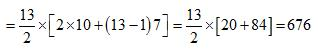Courses

# Test: Divisibility And Remainders- 3

## 5 Questions MCQ Test Quantitative Aptitude for GMAT | Test: Divisibility And Remainders- 3

Description
This mock test of Test: Divisibility And Remainders- 3 for GMAT helps you for every GMAT entrance exam. This contains 5 Multiple Choice Questions for GMAT Test: Divisibility And Remainders- 3 (mcq) to study with solutions a complete question bank. The solved questions answers in this Test: Divisibility And Remainders- 3 quiz give you a good mix of easy questions and tough questions. GMAT students definitely take this Test: Divisibility And Remainders- 3 exercise for a better result in the exam. You can find other Test: Divisibility And Remainders- 3 extra questions, long questions & short questions for GMAT on EduRev as well by searching above.
QUESTION: 1

### Which of these numbers is not divisible by 3?

Solution:

One may answer this question using a calculator and test for divisibility by 3. However we can also test for divisibilty by adding the digits and if the result is divisible by3 then the number is divisible by 3.
3 + 3 + 9 = 15 , divisible by 3.
3 + 4 + 2 = 9 , divisible by 3.
5 + 5 + 2 = 12 , divisible by 3.
1 + 1 + 1 + 1 = 4 , not divisible by 3.
The number 1111 is not divisible by 3 the answer is D.

QUESTION: 2

### What is the smallest positive 2-digit whole number divisible by 3 and such that the sum of its digits is 9?

Solution:

Let xy be the whole number with x and y the two digits that make up the number. The number is divisible by 3 may be written as follows
10 x + y = 3 k
The sum of x and y is equal to 9.
x + y = 9
Solve the above equation for y
y = 9 - x Substitute y = 9 - x in the equation 10 x + y = 3 k to obtain.
10 x + 9 - x = 3 k
Solve for x
x = (k - 3) / 3
x is a positive integer smaller than 10
Let k = 1, 2, 3, ... and select the first value that gives x as an integer. k = 6 gives x = 1
Find y using the equation y = 9 - x = 8
The number we are looking for is 18 and the answer is D. It is divisible by 3 and the sum of its digits is equal to 9 and it is the smallest and positive whole number with such properties.

QUESTION: 3

### Let n! = 1 x 2 x 3 x……….x n for integer n> 1. If p = 1! + (2 x 2!) + (3 x 3!) + ……(10 x 10!),then p+2 when divided by 11! Leaves remainder of

Solution:

If P = 1! = 1
Then P + 2 = 3, when divided by 2! remainder will be 1.
If P = 1! + 2 × 2! = 5
Then, P + 2 = 7 when divided by 3! remainder is still 1.
Hence, P = 1! + (2 × 2!) + (3 × 3!)+ ……+ (10 × 10!)
Hence, when p + 2 is divided by 11!, the remainder is 1.

QUESTION: 4

The remainder, when (1523 + 2323) is divided by 19, is :

Solution:

a+bn is always divisible by a + when n is odd.
Therefore 1523 + 2323 is always divisible by 15 + 23 = 38.
As 38 is a multiple of 19, 1523 + 2323 is divisible by 19.
Therefore,the required remainder is 0.

QUESTION: 5

What is the sum of all two-digit numbers that give a remainder of 3 when they are divided by 7?

Solution:

First of all, we have to identify such 2 digit numbers.
Obviously, they are 10, 17, 24, ….94
The required sum = 10 + 17 … 94.
Now this is an A.P. with a = 10, n = 13 and d = 7
Hence, the sum is